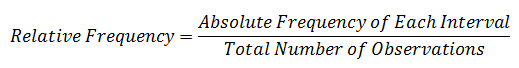# How to Construct a Frequency Distribution

The statistical data that we collect can be presented in the form of a frequency distribution. A frequency distribution refers to summarizing a large data set into a small number of intervals.

Let’s take an example to understand how to construct a frequency distribution. Let’s say we have the following 20 observations with us.

1.5, 2.5, 3, 2.3, 4.3, 5.6, 4.2, 6.7, 5.9, 1.2, 5.4, 9.8, 8.5, 5.5, 2.9, 1.7, 8.8, 6.2, 9.5, 3.8

To construct a frequency distribution for this data, we will follow the following steps.

Step 1: Sort the data in ascending order

The sorted data is presented below:

1.2, 1.5, 1.7, 2.3, 2.5, 2.9, 3, 3.8, 4.2, 4.3, 5.4, 5.5, 5.6, 5.9, 6.2, 6.7, 8.5, 8.8, 9.5, 9.8

Step 2: Calculate the range of data

The range refers to the lower and upper limit for the data intervals. The minimum value is 1.2 and the maximum value is 9.8. This is the range of the data.

Step 3: Decide on the number of intervals in the frequency distribution

This must be done carefully so that the number of intervals is neither to high not too less. If we take very few intervals, our distribution will classify data very broadly. If we take too many intervals, then it won’t really be a summary of data.

In our example, we have 20 observations ranging from 1 to 10. If we use a value interval of 1, then we will have 9 intervals. For 20 values, 9 intervals are too many. So, we can decide to have 5 intervals with a width of 2.

Step 4: Determine the intervals.

Starting form 0, we can have 5 non overlapping intervals as follows:

0 <= r < 2

2 <= r < 4

4 <= r < 6

6 <= r < 8

8 <= r < 10

Note that the above intervals all-encompassing and non-overlapping. Each observation will fall under one (and only one) frequency interval.

Step 5: Tally and count the observations under each interval.

We will now assign each observation to one of these intervals and then count the total number of observations in each interval.

 Interval Absolute Frequency 0 <= r < 2 3 2 <= r < 4 5 4 <= r < 6 6 6 <= r < 8 2 8 <= r < 10 4

Note that the interval 6-8 has the lowest frequency (number of observations) and interval 4-6 has the highest frequency. The above frequency is referred to as Absolute Frequency.

The data in a frequency distribution can also be presented using relative frequencies.Once we have relative frequencies, we can calculate cumulative relative frequencies where as we move from first frequency interval to the last, we keep adding the relative frequencies finally reaching 100%. Cumulative relative frequencies are useful in measuring what fraction of total observations are less than the upper limit of a frequency interval.

We will extend our example to show the relative frequencies and cumulative relative frequencies.

 Interval Absolute Frequency Relative Frequencies Cumulative Relative Frequencies 0 <= r < 2 3 3/20 = 15% 15% 2 <= r < 4 5 5/20 = 25% 40% 4 <= r < 6 6 6/20 = 30% 70% 6 <= r < 8 2 2/20 = 10% 80% 8 <= r < 10 4 4/20 = 20% 100% 20 100%

The cumulative relative frequency is equal to the some of the relative frequencies of all the previous intervals including the current interval. For example, the cumulative absolute frequency for the interval 4 <= r < 6 is 15% + 25% + 30% = 70%.